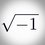# Help: Algebra (Fibonacci Numbers)

The Fibonacci sequence is given by $$F_1 = 1$$, $$F_2 = 1$$, and $$F_{n+1} = F_n + F_{n-1}$$. What is $\sum_{n=4}^\infty \frac{ 1 } { F_{n-3} F_{n+3} } ?$

My work :

\begin{aligned} \sum_{n=4}^\infty \frac{ 1 } { F_{n-3} F_{n+3} } & = \sum_{n=4}^\infty \frac{ 1 } { F_{n-3} F_{n+3} } \times \frac{F_{n+3} - F_{n-3}}{4F_{n}}\\ & = \frac{1}{4} {\left( \sum_{n=4}^\infty \frac{ 1 } { F_{n} F_{n-3} } - \frac{ 1 } { F_{n} F_{n+3} }\right)}\\ & = \frac{1}{4} {\left( \frac{1}{3} + \frac{1}{5} + \frac{1}{16} + 2 \sum_{n=4}^\infty \frac{ 1 } { F_{n} F_{n+3} }\right)}\\ & = \frac{143}{960} + \frac{1}{2} \sum_{n=4}^\infty \frac{ 1 } { F_{n} F_{n+3} }\\ \end{aligned}

Any help will make me happy :)

SOLVEDNote by Jason Chrysoprase
3 years, 10 months ago

This discussion board is a place to discuss our Daily Challenges and the math and science related to those challenges. Explanations are more than just a solution — they should explain the steps and thinking strategies that you used to obtain the solution. Comments should further the discussion of math and science.

When posting on Brilliant:

• Use the emojis to react to an explanation, whether you're congratulating a job well done , or just really confused .
• Ask specific questions about the challenge or the steps in somebody's explanation. Well-posed questions can add a lot to the discussion, but posting "I don't understand!" doesn't help anyone.
• Try to contribute something new to the discussion, whether it is an extension, generalization or other idea related to the challenge.

MarkdownAppears as
*italics* or _italics_ italics
**bold** or __bold__ bold
- bulleted- list
• bulleted
• list
1. numbered2. list
1. numbered
2. list
Note: you must add a full line of space before and after lists for them to show up correctly
paragraph 1paragraph 2

paragraph 1

paragraph 2

[example link](https://brilliant.org)example link
> This is a quote
This is a quote
    # I indented these lines
# 4 spaces, and now they show
# up as a code block.

print "hello world"
# I indented these lines
# 4 spaces, and now they show
# up as a code block.

print "hello world"
MathAppears as
Remember to wrap math in $$ ... $$ or $ ... $ to ensure proper formatting.
2 \times 3 $2 \times 3$
2^{34} $2^{34}$
a_{i-1} $a_{i-1}$
\frac{2}{3} $\frac{2}{3}$
\sqrt{2} $\sqrt{2}$
\sum_{i=1}^3 $\sum_{i=1}^3$
\sin \theta $\sin \theta$
\boxed{123} $\boxed{123}$

Sort by:

Jason, subtract those two sums

$\displaystyle \sum _{ n=4 }^{ \infty }{ \dfrac { 1 }{ { F }_{ n }{ F }_{ n+3 } } } - \displaystyle \sum _{ n=4 }^{ \infty }{ \dfrac { 1 }{ { F }_{ n }{ F }_{ n+3 } } } =0$

$= \dfrac{143}{960}+0$

Interesting excursion into Fibonnaci identities.

- 3 years, 10 months ago

Which two sum ? Which line ?

- 3 years, 10 months ago

Hold on

Okay, remember this line? You're doing a subtraction. How did you end up with an addition?

$= \dfrac{1}{4} \left( \displaystyle \sum_{n=4}^\infty \dfrac{ 1 } { F_{n} F_{n-3} } - \dfrac{ 1 } { F_{n} F_{n+3} }\right)$

From this, you should get

$= \dfrac{1}{4} \left(\dfrac{1}{3}+\dfrac{1}{5}+\dfrac{1}{16}+ \displaystyle \sum_{n=4}^\infty \dfrac{ 1 } { F_{n+3} F_{n} } - \dfrac{ 1 } { F_{n} F_{n+3} }\right)$

Get it now?

- 3 years, 10 months ago